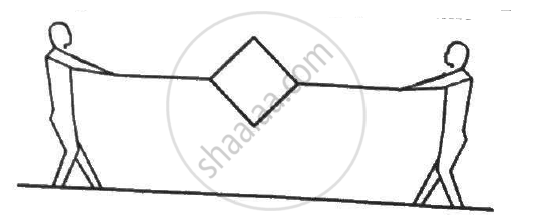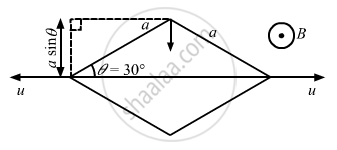Advertisement Remove all ads

# Figure Shows a Metallic Square Frame of Edge A In a Vertical Plane. a Uniform Magnetic Field B Exists in the Space in a Direction Perpendicular to the Plane of the Figure. - Physics

Sum

Figure shows a metallic square frame of edge a in a vertical plane. A uniform magnetic field B exists in the space in a direction perpendicular to the plane of the figure. Two boys pull the opposite corners of the square to deform it into a rhombus. They start pulling the corners at t = 0 and displace the corners at a uniform speed u. (a) Find the induced emf in the frame at the instant when the angles at these corners reduce to 60°. (b) Find the induced current in the frame at this instant if the total resistance of the frame is  R. (c) Find the total charge which flows through a side of the frame by the time the square is deformed into a straight line.Advertisement Remove all ads

#### Solution(a) The effective length of each side is the length that is perpendicular to the velocity of the corners.
Thus, the effective length of each side is a sin θ.
Net effective length for four sides = 4 × a/2 = 2a

∴ Induced emf = Bvl = 2Bau

(b) Current in the frame is given by

$i= \frac{e}{R} = \frac{2auB}{R}$

(c) Total charge q, which flows through the sides of the frame, is given by

$q = \frac{∆ \phi}{R}$

Here,

ΔΦ = Change in the flux

R = Resistance of the coil

$\therefore q = \frac{∆ \phi}{R}$

$= \frac{B( a^2 - 0)}{R}$

$= \frac{B a^2}{R}$

Concept: Induced Emf and Current
Is there an error in this question or solution?
Advertisement Remove all ads

#### APPEARS IN

HC Verma Class 11, Class 12 Concepts of Physics Vol. 2
Chapter 16 Electromagnetic Induction
Q 13 | Page 306
Advertisement Remove all ads

#### Video TutorialsVIEW ALL 

Advertisement Remove all ads
Share
Notifications

View all notifications

Forgot password?